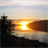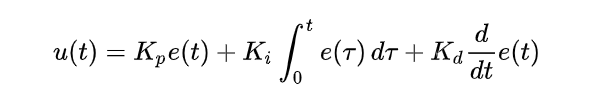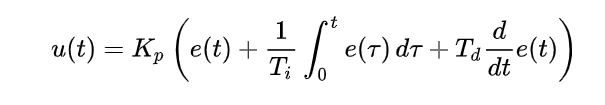# PSoC™ 6 Forum DiscussionsLevel 2
Level 2# Scaling PID loop floating point output to PWM block

Greetings All!

The answer to this question may be painfully obvious, but I'm just not "seeing" it yet.

I'm using a PSOC6 (M4 core with floating point) to close a PID loop on a simple DC-DC boost converter (inner current loop only for now).  I'm setting a desired current value (let's say 1 amp), and I'm measuring the actual current, and I pass the error through the PI loop (for now, my loop is just a PI loop - no derivative gain).

The PWM element I'm using to drive the switching transistor is:

50kHz

PWM counter is 16 bits

period = 1000 counts (so valid compare values are from 0 [0% duty] to 1000 [100% duty])

Center aligned

--> Once I have the output of the PI loop controller (in floating point), how do I scale this to a value of 0 to 1000 for the PWM generation?

1 SolutionLevel 9
Level 9# Re: Scaling PID loop floating point output to PWM block

RiTa,

You have to introduce one more parameter (if there is no such in PID code already),  that is a loop Gain (float). So that

(uint16) PWM duty  = (uint16) ( (float) Gain x (float) PID_output)

Technically, the PI loop needs only 3 parameters (P, I and D), https://en.wikipedia.org/wiki/PID_controller

This is called a Parallel form.but sometimes it is more natural to move out the overall gain outside the equation: gain (Kp), decrement (1/Ti) and delay (Td). This is called a Standard Form./odissey1

3 RepliesLevel 9
Level 9# Re: Scaling PID loop floating point output to PWM block

RiTa,

You have to introduce one more parameter (if there is no such in PID code already),  that is a loop Gain (float). So that

(uint16) PWM duty  = (uint16) ( (float) Gain x (float) PID_output)

Technically, the PI loop needs only 3 parameters (P, I and D), https://en.wikipedia.org/wiki/PID_controller

This is called a Parallel form.but sometimes it is more natural to move out the overall gain outside the equation: gain (Kp), decrement (1/Ti) and delay (Td). This is called a Standard Form./odissey1Level 2
Level 2# Re: Scaling PID loop floating point output to PWM block

Greetings odissey1!

--> Just to make sure I understand your point, an example:

Kp = 1.1f

Ki = 2.2f      (NOT my real PI gain numbers - just an example)

Fsample = 50kHz

Tsample = 20usec

...the next time sample from an equilibrium point [Ierror was 0 before this instant]:

Iref = 1.0A

Iactual = 0.8A

--> Ierror = 0.2A

Iprop_out = 0.2 * 1.1

Iint_out = Iint_out + (0.2 * 2.2 * Tsample)

PID_output = Gain * (Iprop_out + Iint_out)

Where now I have to decide a valid number for "Gain"

--> Do I understand correctly?Level 9
Level 9# Re: Scaling PID loop floating point output to PWM block

RiTa,

Re: PID Controller

I recommend starting with Bret Beauregard's Arduino PID library explanation (#2)

Improving the Beginner’s PID – Introduction « Project Blog

For example, the Gain scaling is discussed in Part 2 of the #1

How to Build a Fixed-Point PI Controller That Just Works: Part II - Jason Sachs

/odissey1